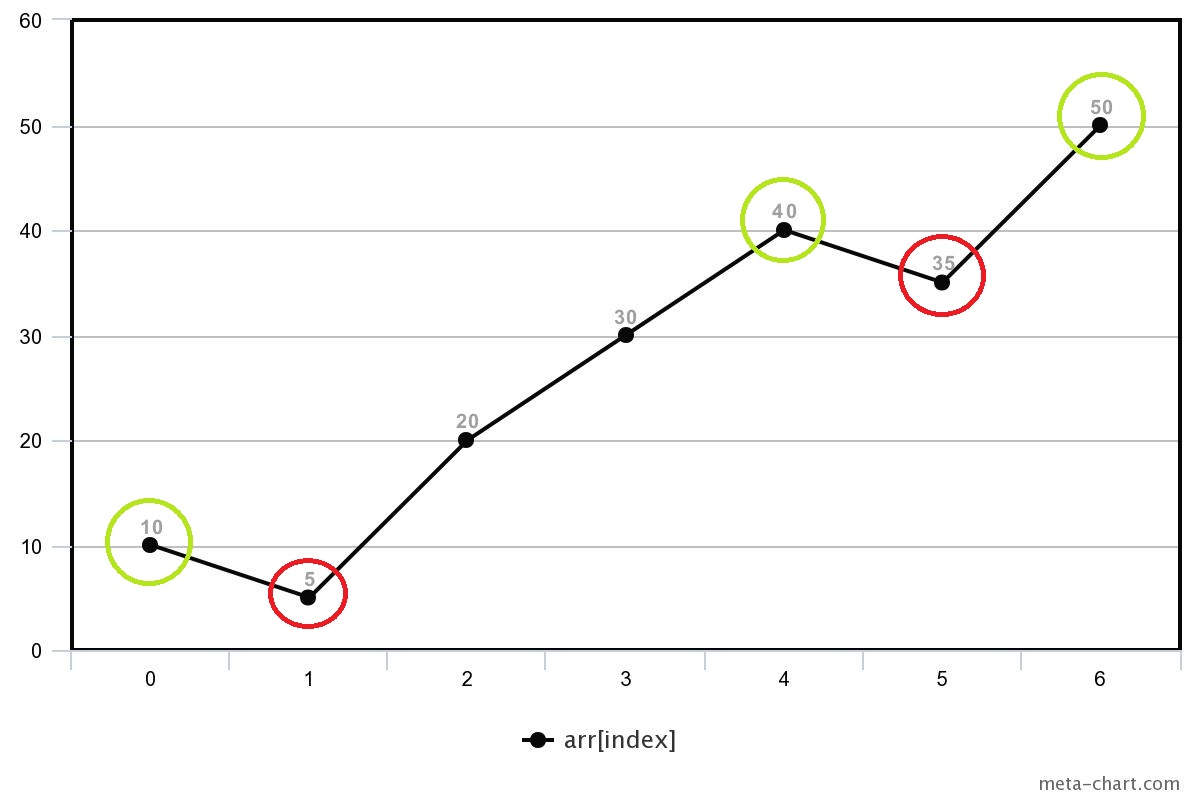2

# Find Indices For Local Minimas and Maximas

Difficulty: EASY
Avg. time to solve
10 min
Success Rate
90%

Problem Statement
Suggest Edit

#### We say that an array element arr[i] is a local minimum if it is less than to its neighbours, and local maximum if it is greater than to its neighbours.

##### Note:
``````For corner elements, we need to consider only one neighbour for comparison.
``````
##### For Example:
``````For the given array :  10 5 20 30 40 35 50
````````````The red circle at index 1,5 are local minima because elements at index 1, and 5 both are smaller to its neighbours.

The green circle at index 0,4,6 are local maxima, because element at index 4 is greater than its neighbours, and element at 0, and 4 are corner elements and also greater than its one neighbour.
``````
##### Input format :
``````The first line of input contains an integer 'T' representing the number of test cases or queries to be processed.

Then the test case follows.

The first line of each test case contains integer N denoting the size of the array.

The second line of each test case contains 'N' single space-separated integers representing the array elements.
``````
##### Output Format:
``````For each test case
In the first line, print all the space-separated indices of local minima in the given array

In the second line, print all the space-separated indices of local maxima in the given array.
``````
##### Note:
``````You do not need to print anything, it has already been taken care of. Just implement the given function.
``````
##### Constraints:
``````1 <= T <= 10
2 <= N <= 10^5
-10^9 <= arr[i] <= 10^9

Time Limit: 1 sec
``````
##### Sample Input 1 :
``````2
3
-10 0 -10
2
4 -2
``````
##### Sample Output 1 :
``````0 2
1
1
0
``````
##### Explanation for sample 1:
``````For the first test case, the element at index 1 is greater than its neighbours (element at index 0, and 2), thus it is the local maxima, and the elements at index 0 and index 2 are corner elements and also smaller than their one neighbours, thus they are the local minima.

For the second test case, the element at index 0 is the corner element and also greater than its one neighbour, thus it is local maxima, and the element at index 1 is also the corner element and also smaller than its one neighbour thus it is local minima.
``````
##### Sample Input 2 :
``````2
7
10 5 20 30 40 35 50
3
1 2 2
``````
##### Sample Output 2 :
``````1 5
0 4 6
0
-1
``````Console